# Percent error

Here you will learn about percent error, including how to calculate it and use it to solve problems.

Students first learn about percent error in the 7 th grade and expand this knowledge as they move through middle school and high school statistics.

## What is percent error?

The percent error is a type of percent problem that describes the difference between an estimated value or observed value and an actual value, or accepted value, or real value as a percent of the actual value. In other words, percent error is how big your error is when you estimate a measurement.

The way you calculate a percent error is similar to how you calculate a percent change (percentage change).

To calculate the percent error you can apply the following formula:

The difference in values is the positive difference between the estimated value and the actual value (or the absolute value of the error). This is also known as the absolute error.

The ratio of the difference in values to the actual value,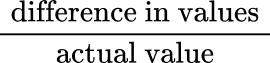, is known as the relative error.

Let’s look at an example.

Lucas estimated the weight of his pet rabbit, Blu, to be 5.5 pounds. Blu’s actual weight is 4.75 pounds. Find the percent error.

\begin{aligned}& \text {estimated weight }=5.5 \; pounds \\\\ & \text {actual weight }=4.75 \; pounds \\\\ & \text {Difference in values also known as the absolute error: } 5.5 - 4.75=0.75 \end{aligned}

Using the formula,

Lucas’s percent error is 15.8 \% meaning his estimate was about 15.8 \% off from the actual weight measurement.

### What is percent error?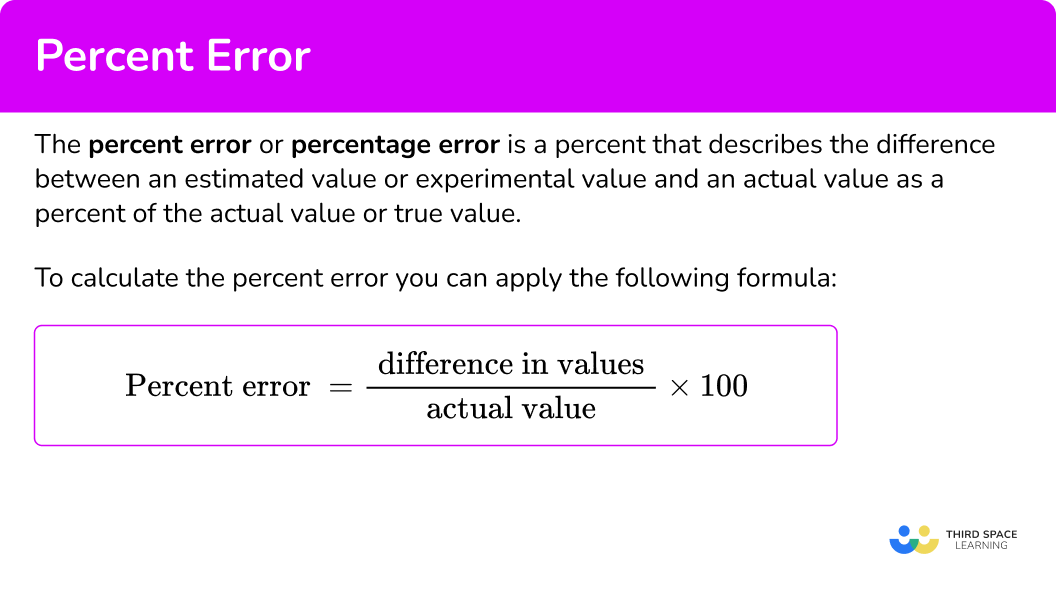## Common Core State Standards

How does this apply to 7 th grade math?

• Grade 7 – Ratios and Proportional Relationships (7.RP.A.3)
Use proportional relationships to solve multistep ratio and percent problems. Examples: simple interest, tax, markups and markdowns, gratuities and commissions, fees, percent increase and decrease, percent error

## How to find percent error

In order to find the percent error:

1. Find the positive difference between the actual value and the estimated value.
2. Use the formula to calculate.

## Percent error examples

### Example 1: finding percent error

Elba estimated that 190 people are going to attend the spring concert. The actual number of people attending was 214. Find the percent error.

1. Find the positive difference between the actual value and the estimated value.

\begin{aligned}& \text {Estimated value }=190 \\\\ & \text {Actual value }=214 \\\\ & \text {Difference in values: } 214-190=24 \end{aligned}

2Use the formula to calculate.

(you may have to round the division to three decimal places)

The percent error is 11.2 \%

### Example 2: finding percent error

If the actual value is 74 and the estimated value is 70, what is the percent error?

Find the positive difference between the actual value and the estimated value.

Use the formula to calculate.

### Example 3: finding percent error

Sophia is working at a vet clinic. She estimated the weight of a cat to be 10 pounds. When she weighed the cat, the actual weight was 8.5 pounds. What is the percent error?

Find the positive difference between the actual value and the estimated value.

Use the formula to calculate.

### Example 4: finding percent error

Ryan thinks the cost of a video game is \$65. However, the actual cost is \$ 58. What is the percent error?

Find the positive difference between the actual value and the estimated value.

Use the formula to calculate.

### Example 5: finding percent error

Johnny estimated the population of his small town to be 3,225 people. The actual population of his town is 4,057. What is the percent error?

Find the positive difference between the actual value and the estimated value.

Use the formula to calculate.

Ginny wants to buy a new gaming device for her nephew. She estimates the cost to be \$250. If the percent error is 8 \% above the actual value, what is the actual price of the device? (round to the nearest hundredth). Find the positive difference between the actual value and the estimated value. Use the formula to calculate. ### Teaching tips for percent error • Incorporate discovery based learning activities so students can develop the formula for finding percent error. • Although worksheets have their place, consider using real world examples that are relevant to students to find percent error or percent change. • Students have access to percent error calculators online. However, having them demonstrate and explain their work will build understanding as opposed to just typing numbers into a digital calculator. ### Easy mistakes to make • Forgetting to multiply the answer by \textbf{100} Finding percent error means to actually find a percent. After doing the calculations of finding the difference between the values and then dividing by the actual value, students forget to multiply by 100. For example, find the percent error if the estimated amount is 56 and the actual amount is 68.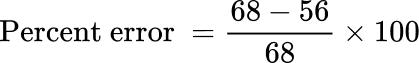\text {Percent error }=0.176 — cannot leave the answer as a decimal. The answer must be converted to a percent, so multiplying by 100 is essential. \text {Percent error }=0.176 × 100=17.6 \% • Finding the negative difference When finding percent error, it technically does not matter which way you find the difference. For example, finding the percent error between an estimated value of 14 and an actual value of 19. You can find the difference by: 19-14 OR 14-19 , in either case, be sure to take the absolute value because it’s the positive difference between the values. ### Practice percent error questions 1. Tim estimated the weight of his dog to be 20 pounds. When he took his dog to the vet, the dog weighed 18 pounds. What is the percent error? 0.11 \%1.11 \%11.1 \%12 \%To find percent error, first find the positive difference between the estimated value and the actual value. 20-18=2 Then use the formula to calculate.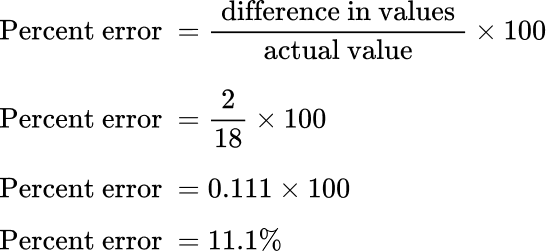2. Billy wants to buy a dozen doughnuts for his soccer team. He estimates that it will be \$ 15. The actual cost of the dozen doughnuts is \\$ 16.25. What is Billy’s percent error?

0.769 \%6.9 \%7.9 \%7.69 \%Find the positive difference between the estimated value and the actual value.

16.25-15=1.25

Then use the formula to calculate.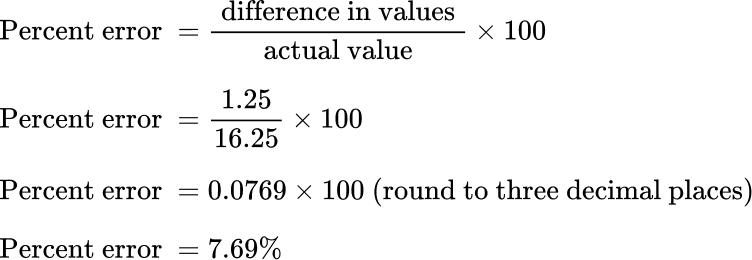3. The principal of Lakewood Heights High School estimated that 380 people will come to the championship game. There were actually 432 people at the game. What is the principal’s percent error?

0.012 \%1.20 \%12 \%0.12 \%Find the positive difference between the estimated value and the actual value.

432-380=52

Then use the percent error formula to calculate.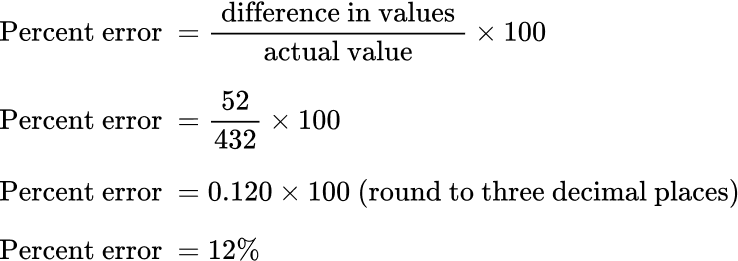4. Lena planted a tomato plant. She estimated that the plant would be 7 inches after the first month. The plant was actually 9.9 inches after the first month. What is the percent error?

0.293 \%29.3 \%2.93 \%293 \%Find the positive difference between the values.

9.9-7=2.9

Then use the percent error formula to calculate.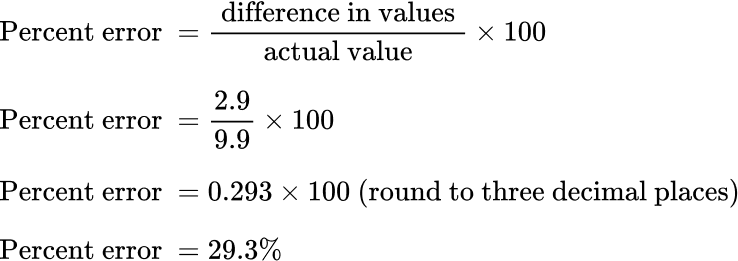Lena’s percent error is 29.3 \%

5. Kyle estimated he would run 15 miles this week. He actually ran 33 miles. What is the percent error?

0.545 \%5.45 \%545 \%54.5 \%Find the positive difference between the values.

33-15=18

Then use the percent error formula to calculate.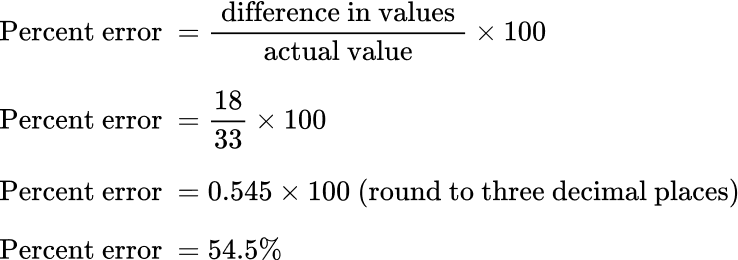Kyle’s percent error is 54.5 \%.

6. Kelly estimated that she would score 30 points at her basketball game. If her percent of error is 20 \% above the actual amount, what is the actual amount of points she scored in the game?

25 \, points26 \, points30 \, points20 \, pointsThe estimated value is 30 points. The actual value is unknown, label it as x.

The percent error is 20 \%

Use the formula to calculate.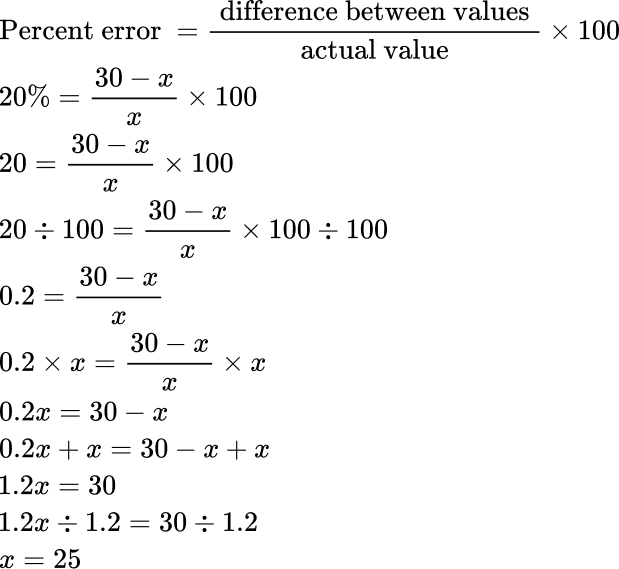Kelly actually scored 25 points in the game.

## Percent error FAQs

Can a decimal number be a percent error?

When calculating percent error, you will get a decimal number before converting it to a percent.

Can a percent error be negative?

Sometimes a negative sign is used to describe a percent error when the estimated value is less than the actual value, making the percent a negative value.

Is there a calculator that can quickly find the percent error?

Yes, there are digital calculators online that can do quick calculations such as a standard deviation calculator, a percent error calculator, and a percent calculator (percentage calculator). Although technology is there to help us, be sure to understand what the calculations mean.

## Still stuck?

At Third Space Learning, we specialize in helping teachers and school leaders to provide personalized math support for more of their students through high-quality, online one-on-one math tutoring delivered by subject experts.

Each week, our tutors support thousands of students who are at risk of not meeting their grade-level expectations, and help accelerate their progress and boost their confidence.